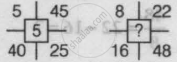# Find the Missing Character in The Following Questions. - Logical Reasoning

MCQ

Find the missing character in the following question.• 5

• 6

• 12

• 9

#### Solution

6
Explanation:
Here, in the first figure,
25/5=45-40

= 5
5 = 5 = 5
Similarly, in the second figure,
48/8=22-16=?

6 = 6 = ?
So, 6 = 6 = 6

Concept: Inserting the Missing Character (Entrance Exam)
Is there an error in this question or solution?